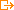# What is the complexity of the algorithm

## Time complexity and O notation (see also Wikipedia:Time complexity,Space complexity,Landau symbol)

Usually it is difficult or impossible to give the exact equation for the time T (n) for an algorithm. One tries to estimate the effort asymptotically, i. H. one tries to find as simple a function as possible, which limits the function of the expenditure of time for large n. It should therefore apply that T (n) ≤ c · f (n) applies from a certain element n0, where c is a positive constant. This estimate is also referred to as the asymptotic order O (f).

Here is the exact, mathematical formulation of the asymptotic order: O (f) = {T | T: N R.0+ and ∃c ∈ R.+ ∃n0 N ∀n ≥ n0: T (n) ≤ c · f (n)}

Example:

The function of the expenditure of time T (n) = 3n is given2 - 6n + 9 Which function can be used as an estimate?

T (n) is a quadratic function, which in turn has a quadratic function, namely f (n) = n2 should be approached asymptotically.
So T (n) ≤ c · f (n), i.e. 3n2 - 6n + 9 ≤ c n2 be valid. If one sets c = 3, the equation can be transformed in an elementary way and one obtains:For all n ≥ 1.5 the expression 1.5 · n is restricted2 the function T (n). Thus for the function T (n) ∈ O (n2).

Accordingly, polynomial algorithms of degree k always have a runtime cost of O (nk), exponential algorithms require the effort O (2n), O (n!) Or O (nn).